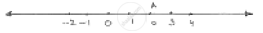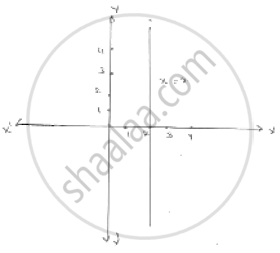# Give the Geometric Representation of the Following Equation (A) on the Number Line (B) on the Cartesian Plane : X = 2 - Mathematics

Give the geometric representation of the following equation
(a) on the number line   (b) on the Cartesian plane :  x = 2

#### Solutionx = 2
Point A represents  x = 2 number line
On Cartesian plane, equation represents all points on  y - axis for which  x = 2

Concept: Equations of Lines Parallel to the X-axis and Y-axis
Is there an error in this question or solution?

#### APPEARS IN

RD Sharma Mathematics for Class 9
Chapter 7 Linear Equations in Two Variables
Exercise 7.4 | Q 1.1

Share